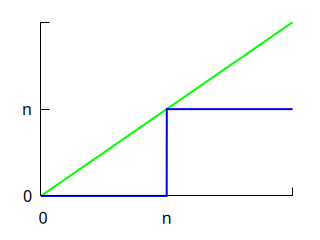# The Markov and Chebyshev Inequalities

We intuitively feel it is rare for an observation to deviate greatly from the expected value. Markov’s inequality and Chebyshev’s inequality place this intuition on firm mathematical ground.

I use the following graph to remember them.Here, $$n$$ is some positive number. The blue line (the function that takes the value $$0$$ for all inputs below $$n$$, and $$n$$ otherwise) always lies under the green line (the identity function).

## Markov’s Inequality

Suppose $$n$$ is a positive integer. Since the blue line lies under the green line:

$0 + ... + 0 + n + n + ... \le 0 + 1 + 2 + ...$

Suppose the random variable $$X$$ takes nonnegative integer values. Let $$p(i)$$ be the probability of $$i$$ occuring, and multiply the $$i$$th term by $$p(i)$$:

$0(p(0) + ... + p(n-1)) + n(p(n) + p(n+1) + ... ) \le 0 p(0) + 1 p(1) + 2 p(2) + ...$

In other words, we have Markov’s inequality:

$n \Pr[X \ge n] \le E[X]$

The graph captures this inequality, and also makes it clear why equality is attained only when $$p(i) = 0$$ for all $$i \ne 0, n$$ (the only two points where the two functions agree).

The argument generalizes to any random variable that takes nonnegative values.

## Chebyshev’s Inequality

We start from Markov’s inequality:

$\Pr[X \ge n] \le E[X] / n$

Then we complain about the $$n$$ in the denominator. The bound is so weak. Can’t we do better than a denominator that increases only linearly? Why not at least quadratically? Why not $$n^2$$?

No problem! We simply square everything:

$\Pr[X^2 \ge n^2] \le E[X^2] / n^2$

[Actually, we cannot square quantities arbitrarily. More precisely, we have replaced $$n$$ by $$n^2$$, and are now considering the random variable $$X^2$$ instead of $$X$$.]

But nobody likes $$E[X^2]$$; everybody prefers the related $$E[(X - E[X])^2]$$, aka the variance. In general, we prefer central moments to raw moments because they describe our data independently of translation.

Thus we replace $$X$$ by $$X - E[X]$$ to get Chebyshev’s Inequality:

$\Pr[(X - E[X])^2 \ge n^2] = \Pr[|X-E[X]|\ge n] \le E[(X - E[X])^2] / n^2$

We let $$\mu = E[X]$$ and $$\sigma = \sqrt{E[(X-E[X])^2]}$$, and substitute $$n$$ with $$\sigma n$$ so it looks better:

$\Pr[|X-\mu|\ge \sigma n] \le 1 / n^2$

We can remove the restriction that $$X$$ is nonnegative: the random variables $$X^2$$ and $$|X - \mu|$$ are nonnegative even if $$X$$ is not.

## Weak Law of Large Numbers

Let $$X_1,...,X_N$$ be independent random variables with the same mean $$\mu$$ and same variance $$\sigma^2$$. Define a new random variable:

$X = \frac{X_1 + ... + X_N}{N}$

Then:

$\Pr[|X - \mu| \ge \alpha] \le \frac {\sigma^2 }{ \alpha^2 N}$

Proof outline: Show $$E(X) = \mu$$ and $$\operatorname{var}(X) = \sigma^2 / N$$ then apply Chebyshev’s inequality.

## Higher Moments

A similar argument works for any power $$m$$ higher than 2. None of them are named after anyone, as far as I know. For any random variable $$X$$ and positive $$n$$, we have:

$\Pr[|X| \ge n] \le E[|X|^m] / n^m$

and:

$\Pr[|X-\mu| \ge n] \le E[|X-\mu|^m] / n^m$

## An Exponential Improvement

Under some conditions, we can get a denominator that increases exponentially. In other words, large values of $$\Pr[|X-\mu|]$$ are almost impossible. See the Chernoff bound.

Ben Lynn blynn@cs.stanford.edu 💡